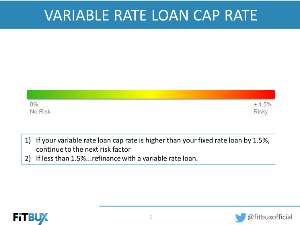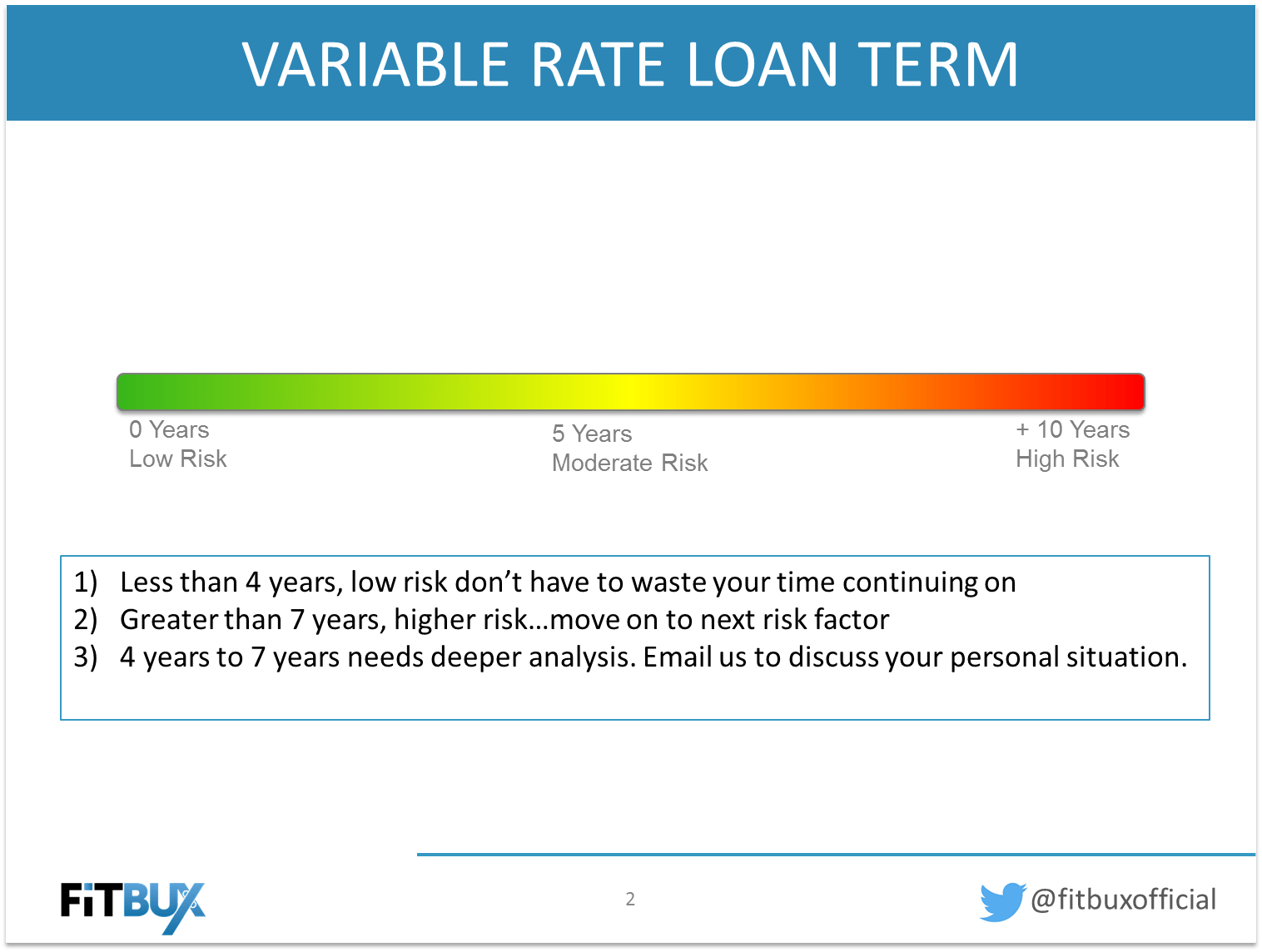# 4 Steps In Deciding Variable vs Fixed Rate Student Loan Refinancing

February 21, 2019

By Joseph Reinke, CFA, Founder of FitBUX

When we refinance our student loans, we ask ourselves one simple question: Does refinancing save me money? The marketing geniuses at lending companies know this. That is why you see student loan refinance advertisements with big bold words saying how much an average person saves.

However, as we point out in our Ultimate Student Loan Refinance Guide, the decision is not that simple. This especially holds true when considering variable vs fixed rate student loans.

Determining when to refinance using a variable rate student loan is a complex topic.  We at FitBUX have constructed this easy to understand guide. This guide will take you through four risk factors that assist you in answering the question: “Is refinancing with a variable rate student loan the right choice for me?”

Also, to help you, we developed a step-by-step student loan refinance checklist.  Complete the info below and we’ll send you the checklist via email!

Below are the four risk factors and accompanying videos.

## Risk Factor 1: Current Variable vs. Fixed Rate Student Loans

This is the easiest risk factor to analyze. If the proposed interest rate on the variable rate student loan is greater than your fixed interest rate student loan, then keep your fixed rate loan and go enjoy the rest of the day. Simply put, in today’s interest rate environment, rates will most likely go up from where they are today.  There is no upside in sight for you under that scenario.

If the starting interest rate offered on the variable rate loan is lower than the rate of your current fixed-rate loan, you should proceed to the second risk factor below.

## Risk Factor 2: What is the Cap Rate?

All variable rate student loans have interest rates that move up and down.  Most do so on a monthly or annual basis. The Cap Rate is simply the highest interest that you can be charged over the life of the loan. For example, if the Cap Rate is 9.0%, then the highest interest rate you can be charged is 9.0%.

The Cap Rate is important because variable rate monthly payments start off being lower than your fixed rate payments. However, if interest rates rise your monthly payment under a variable rate student loan could end up being greater than it would be under your current fixed rate loan.

When evaluating your Cap Rate, there is no “magic” number. For example, one simply can not say “9.0% is too high. Therefore, I should not refinance with a variable rate student loan if the cap rate is 9.0%.”  Instead, you must look at the Cap Rate relative to your fixed interest rate.

As a general guideline, if the Cap Rate on the variable rate student loan you are being offered is greater than your fixed rate loan by 1.5% or less, then refinancing into the variable rate student loan would make sense. For example, if your fixed interest rate is 6.0% and the cap rate is 7.5% or lower, then refinance into a variable rate student loan.

If, using our example, the cap rate is greater than 7.5% then the variable rate student loan has a high amount of risk and you need to proceed to the third risk factor below.## Risk Factor 3: What is the Loan Term?

The third risk factor to consider when contemplating refinancing with a variable rate student loan is the term.  Is your variable rate student loan 2 years, 5 years, 10 years, etc..?

Historically, interest have tended to go up slowly.  Therefore, the shorter the loan term, the less likely the interest rate is going to increase dramatically. Thus, if you have a short-term loan, your interest rate is less likely to increase to the Cap Rate.

In general, a variable rate student loan with a term of less than 4 years is considered low risk, all else being. This also applies if you plan on paying off the loan in less than 4 years. Conversely, a variable rate loan with a term greater than 7 years is considered high risk and you will need to analyze the last risk factor below.

If you are offered a variable rate student loan with a term between 4-7 years, a deeper analysis must be performed and we recommend contacting us for a free student loan analysis.## Risk Factor 4: What is the Student Loan Balance?

The last risk factor to analyze when determining if you should use a variable rate student loan to refinance is the size of the loan.

Let’s assume you owe \$1,000 on a variable rate student loan and the current interest rate on that loan is 2% and the Cap Rate is 8%.

Let’s pretend that the interest rate on the loan changes overnight from 2-8%. What would happen to my monthly payment? The required monthly payment only goes up by \$2.75.  On the other hand, if the amount you owed was \$100,000, the monthly payment would increase by \$274 a month.

Therefore, the lower your student loan balance is, the lower amount of risk you are exposed to when considering a variable rate student loan.  Determining what loan balance is appropriate highly depends on your income level and how quickly you plan on repaying your loans.

In general, if the above risk factors are low and you plan on paying off your loans quickly, you may want to consider refinancing 100% of your loans into a variable rate loan.

If the above risk factors are considered moderate risk, you may want to limit your exposure to variable rate loans at approximately 50% of your income.  I.e. if you make \$100,000 per year limit your variable loan balance to \$50,000 or less.

If the above risk factors are high risk then you may want to avoid variable rate loans altogether.  If you do want to use them we would not recommend more than 10%-20% of your annual income, i.e. you make \$100,000 per year your variable rate loan balance would be less than \$20,000.Of course, each situation is different.  If you would like us to take a look at your personal situation, let us know. Sign up at Fitbux.com today and use our one-of-a-kind technology to evaluate your situation. Its’ free….

Current Variable Interest Rates:

5 Year: 2.7%
10 Year: 4.9%
15 Year: 5.28%
20 Year: 5.53%

Also, if you’d like to read more about managing your money more efficiently, check out our main blog page.  For student loan refinance articles check out this page.FitBUX student loan help, Student Loan Refinance

Never miss important financial information!

Subscribe to our monthly newsletter to keep up with the latest trends!

• […]  The cap rate on the proposed variable rate student loan is the second risk factor you must consider when contemplating using a variable rate loan to refinance your student loan. This video is best used in conjunction with the following guide….http://fitbu.. […]